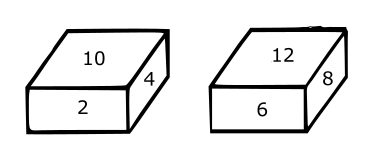0
10382

# Open dice Questions for SSC CGL PDF

Download SSC CGL Open dice Questions with answers PDF based on previous papers very useful for SSC CGL exams. Top-10 Very Important Open dice Questions for SSC Exams.

Question 1:

a)

b)

c)

d)

Question 2: A paper sheet is made into the following pattern (A). Find the cube that will best represent after folding the paper (A).

a) 1, 2 and 3 only

b) 1, 3 and 4 only

c) 2, 3 and 4 only

d) 2 and 3 only

Question 3: Four different positions of the same dice are shown. Find the number on the face opposite to the one having 3.

a) 2

b) 4

c) 1

d) 6

Question 4: Four positions of a cube are shown below. Which color is opposite to white color in the given cubes?

a) Orange

b) Blue

c) Red

d) Yellow

e) None of the above

Question 5: Four positions of a dice are given below:

Find the number on the face opposite to the face showing 4.

a) 5

b) 3

c) 6

d) 1

Question 6: From the given blocks when 10 is at the bottom, which number will be at the top ?a) 8

b) 12

c) 6

d) 4

Question 7: From the given options which answer figure can be formed by folding the figure given in the question

a)

b)

c)

d)

Question 8: From the given options, which answer figure can be formed by folding the figure given in the question ?

a)

b)

c)

d)

Question 9: From the given options, which answer figure can be formed by folding the figure given in the question ?

a)

b)

c)

d)

Question 10: From the given options, which answer figure can be formed by folding the figure given in the question ?

a)

b)

c)

d)

Question 11: From the given options, which answer figure can be formed by folding the figure given in the question ?

a)

b)

c)

d)

Question 12: From the given options, which answer figure can be formed by folding the figure given in the question ?

a)

b)

c)

d)

Question 13: From the given options, which answer figure can be formed by folding the figure given in the question ?

a)

b)

c)

d)

Question 14: From the given options, which answer figure can be formed by folding the figure in the question ?

a)

b)

c)

d)

Question 15: From the given options, which figure can be formed by folding the figure given in the question ?

a)

b)

c)

d)

2, 3 and 4 will best represent after folding the paper (A).

From 4 figure of dice we conclude that adjacent of 3 is 4,5,6,1, so 2 will be opposit of 3.

When we fold the question figure, we get ‘$’ as the base of the cube and ‘|’ as top, thus they face each other. Also, ‘%’ will face ‘>’ and ‘*’ will face ‘#’. Thus, (*,#) , ($,|) and (%,>) cannot be the adjacent sides of the cube as mentioned in the last three options respectively.

=> Ans – (A)